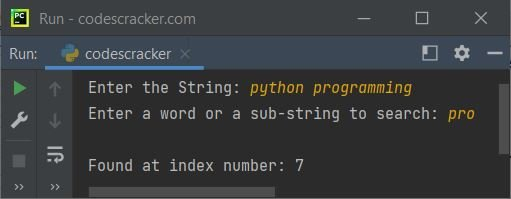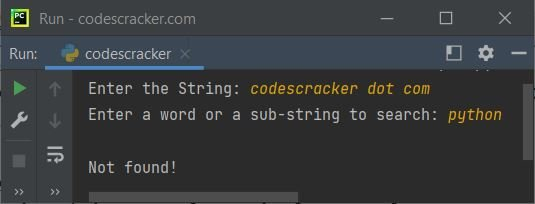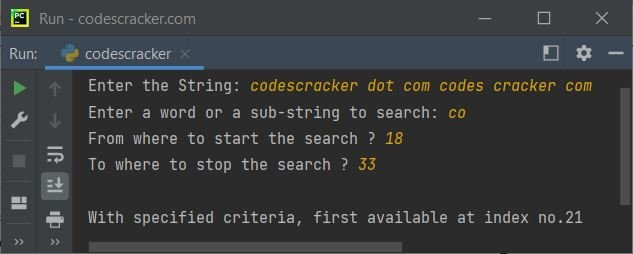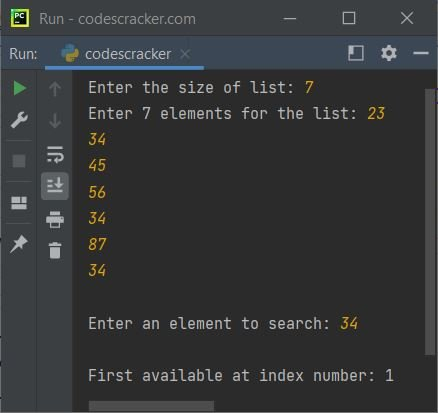# Python index() Function

The index() function in Python returns the index number of the first occurrence of a specified value in a list or string. For example:

```mylist = [13, 43, 54, 43, 65]
print(mylist.index(43))

mystring = "codescracker dot com"
print(mystring.index("co"))```

The output produced by above Python program, demonstrating the index() function is:

```1
0```

From above program, the statement:

`print(mylist.index(43))`

prints the index number of the value 43 in the list named mylist. Since the first occurrence of 43 is at index number one. Therefore the above statement prints 1 on output. Whereas the following statement:

`print(mystring.index("co"))`

searched the sub-string co in the string mystring or codescracker dot com. And because, the first occurrence of co is available at 0th index of the string. Therefore the output is 0.

## Python index() Function Syntax

The syntax to use the index() function in Python is:

`mystring.index(value, start, end)`

The value refers to the value (element or sub-string) that is going to be search , start tells from where to start the search, end tells where to stop the search.

The start and end both parameters are optional. The default value of start is 0, whereas the default value of end is the length of the list or string.

#### What if Specified Value is Not Available in the List or String ?

In case, if the specified value is available in the list or string, the index() function returns or raises an exception named ValueError. Therefore to handle this exception, the function index() needs to be wrapped inside a try block to catch the raised exception using except block. An example program that shows how to handle this type of error, is given below.

## Python index() Example - For String

Here is an example of index() function. This program demonstrates the function, that how we can search and find the position of a sub-string in a string, entered by user:

```print("Enter the String: ", end="")
str = input()
print("Enter a word or a sub-string to search: ", end="")
s = input()

pos = str.index(s)
print("\nFound at index number:", pos)```

The snapshot given below shows the sample run of above Python program, with user input python programming as string and pro as sub-string to search and print its index:Here is another example of index() function used to search a sub-string in a given string. This program handles the exception raised by index(), when the specified value would be unavailable.

```print("Enter the String: ", end="")
str = input()
print("Enter a word or a sub-string to search: ", end="")
s = input()

try:
pos = str.index(s)
print("\nFound at index number:", pos)
except ValueError:

Here is its sample run with user input codescracker dot com as string and python as sub-string to search:Here is one more example uses index() function with all its parameters:

```print("Enter the String: ", end="")
str = input()
print("Enter a word or a sub-string to search: ", end="")
s = input()
print("From where to start the search ? ", end="")
start = int(input())
print("To where to stop the search ? ", end="")
end = int(input())

if start > len(str) or end > len(str):
print("\nInvalid Input!")
elif start > end:
print("\nInvalid Input!")
else:
try:
pos = str.index(s, start, end)
print("\nWith specified criteria, first available at index no.", pos, sep="")
except ValueError:

The sample run with user input codescracker dot com codes cracker com as string, co as sub-string to search, 18 as index to start with, 33 as index to stop at, is shown in the snapshot given below:## Python index() Example - For List

This is the last example program of this article, created to demonstrate the use of index() function while working with list in Python:

```print("Enter the size of list: ", end="")
tot = int(input())
print("Enter", tot, "elements for the list: ", end="")
arr = []
for i in range(tot):
val = input()
arr.append(val)

print("\nEnter an element to search: ", end="")
element = input()
try:
pos = arr.index(element)
print("\nFirst available at index number:", pos)
except ValueError:
print("\nNot available in the list!")```

Sample run with user input 4 as size of list, 23, 34, 45, 56, 34, 87, 34 as its four element and 34 as element to search and print its index, is shown in the snapshot given below:Note: Index numbering always starts with 0.

You can also use the two optional arguments when you need while working with list, in the same way as shown in the previous program, that is the last example program of index() function for string section.

Python Online Test

« Previous Function Next Function »